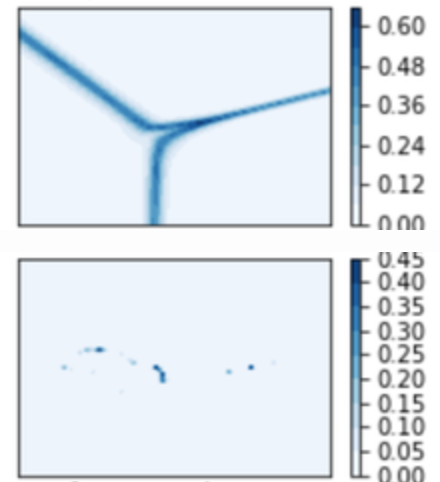# Strange behaviour of controuf when plotting contours on the same model

Hi folks, I’m observing some strange behaviour when using `plt.contourf` to plot contours. In the first instance everything seems fine. But when I plot again the contours are barely visible.

(e.g.)Each plot corresponds to the same underlying model for which the contours are derived, but different way of initialising its params. The only thing that jumps immediately is that the second plot has max values around 0.45 while the first 0.60, from the colorbar. Could that be the issue here though?

`MWE:`

``````def plot_contour(points, z, title, levels=14, ax=None, fig=None):
N = np.int_(np.sqrt(points.shape))
z = z.reshape((N, N))
x = np.linspace(np.min(points[:, 0]), np.max(points[:, 0]), N)
y = np.linspace(np.min(points[:, 1]), np.max(points[:, 1]), N)

if ax is None or fig is None:
plt.figure()
cs = plt.contourf(x, y, z, levels=levels, cmap='Blues')
cbar = plt.colorbar(cs)
plt.suptitle(title)
else:
cs = ax.contourf(x, y, z, levels=levels, cmap='Blues')
cbar = fig.colorbar(cs, ax=ax)
ax.set_title(title)
``````

Suggest you not use contour at first until you better understand your data. You are also allowing automatic levels (levels=14) which can be problematic. Try specifying an array of contours.

MWE:

That is not a working example`levels=np.array(0, 1, 0.01)` with numbers tuned to your data…